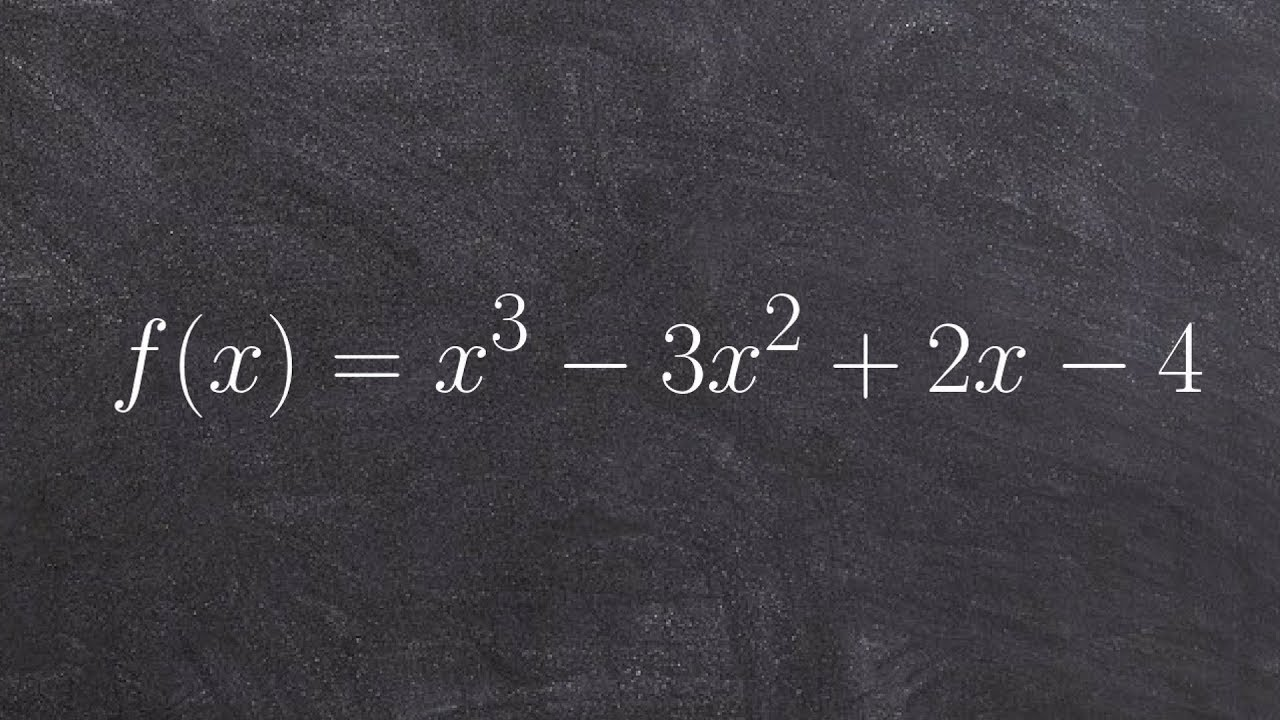# How to apply the remainder theorem by evaluating and synthetic division👉 Learn about and how to apply the remainder and factor theorem. The remainder theorem states that f(a) is the remainder when the polynomial f(x) is divided by x - a. Thus, given a polynomial, f(x), which is to be divided by a linear binomial of form x - a, the remainder of the division is given by f(a).

The factor theorem is a special case of the remainder theorem which states that if f(a) = 0, then x - a is a factor of the polynomial f(x). Thus, given a polynomial, f(x), to see if a linear binomial of form x - a is a factor of the polynomial, we solve for f(a). If f(a) = 0, then x - a is a factor, and x - a is not a factor otherwise.

These two theorems help us understand how we better understand the relationship between a polynomial function, its factors, and the remainder. Using the theorem also can save us time from determining if we have a factor or zero of a polynomial without having to use division.

👏SUBSCRIBE to my channel here: https://www.youtube.com/user/mrbrianmclogan?sub_confirmation=1

❤️Support my channel by becoming a member: https://www.youtube.com/channel/UCQv3dpUXUWvDFQarHrS5P9A/join

Organized Videos:
✅Remainder and Factor Theorem
✅Remainder and Factor Theorem | Learn About
✅How to apply the Remainder and Factor Theorem

🗂️ Organized playlists by classes here: https://www.youtube.com/user/MrBrianMcLogan/playlists

🌐 My Website - http://www.freemathvideos.com

🎯Survive Math Class Checklist: Ten Steps to a Better Year: https://www.brianmclogan.com/email-capture-fdea604e-9ee8-433f-aa93-c6fefdfe4d57

Connect with me:
⚡️Instagram - https://www.instagram.com/brianmclogan/

👨‍🏫 Current Courses on Udemy: https://www.udemy.com/user/brianmclogan2/

👨‍👩‍👧‍👧 About Me: I make short, to-the-point online math tutorials. I struggled with math growing up and have been able to use those experiences to help students improve in math through practical applications and tips. Find more here: https://www.freemathvideos.com/about-me/

#polynomials #brianmclogan